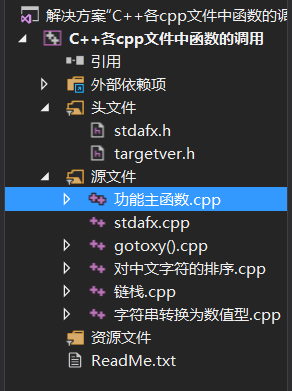# C++ 一个函数调用其他.cpp文件中的函数

【 示例 】

• ### 首先是资源管理窗口：• 功能主函数.cpp
// C++上机作业.cpp : 定义控制台应用程序的入口点。
//'0-9': 48-57

#include "stdafx.h"
using namespace std;
extern void gotoxy(short x, short y);
extern void sort_by_name();
extern int Strtoint();

int main()
{
system("title 功能主函数");
gotoxy(23, 2); cout << "功能列表";
gotoxy(15, 3); cout << "1:字符串转换为数值类型";
gotoxy(15, 4); cout << "2:对中文字符进行排序";

gotoxy(0, 10);
int choice = 0;
cout << "请输入您要执行的功能：";
cin >> choice;
getchar();    //吸收回车
switch (choice)
{
case 1:
Strtoint();
break;
case 2:
sort_by_name();
break;
default:
cout << "选择失败，感谢使用，再见！" << endl << endl;
}
return 0;
}

• stdafx.h（stdandard application framework extensions
// stdafx.h : 标准系统包含文件的包含文件，
// 或是经常使用但不常更改的
// 特定于项目的包含文件
//

#pragma once

#include "targetver.h"

#include <stdio.h>
#include <tchar.h>
#include <iostream>
#include <Windows.h>
#include <string>    //注意这里的string与cstring中的使用差别，在定义与使用cout输出string类型字符串的时候，最好使用string库，否则可能会出现乱码以及错误等一系列错误

// TODO:  在此处引用程序需要的其他头文件

• gotoxy().cpp
#include "stdafx.h"
using namespace std;

void gotoxy(short x, short y)
{
COORD pos = { x,y };
HANDLE hOut = GetStdHandle(STD_OUTPUT_HANDLE);
SetConsoleCursorPosition(hOut, pos);
}
• 对中文字符的排序.cpp
//对中文字符串进行排序时，默认是按照第一个字符的第一个拼音的顺序进行排序

#include "stdafx.h"
using namespace std;

void sort_by_name()
{
string s = { "一号","二号","三号","四号" }, t;
for (int i = 0; i<4; i++)
{
for (int j = i; j<4; j++)
{
if (s[i]>s[j])
{
t = s[i];
s[i] = s[j];
s[j] = t;
}
}
}
for (int i = 0; i < 4; i++)
{
cout << s[i] << endl;
}
cout << "功能运行结束！" << endl << endl;
}
• 字符串转换为数值型.cpp
#include "stdafx.h"
using namespace std;

int Strtoint_0(const char str[])  //字符串数字转换为整形
{
int i = 0, j = 0;
long long number1 = 0;    //定义一个长整形变量，用来存储转换后得到的值
int number = { 0 };    //定义一个数组，用来存储转换后得到的值
int symbol = 1;    //符号常量，0为负，1为正(默认为正)
while (str[i] != '\0')	  //测试输出判断是否正确
{
while (str[i] == ' ')
{
i++;
}
if ((str[i] == '+' || str[i] == '-'))
{
i++;
if (str[i] == '-')
{
symbol = 0;
}
}
else if (str[i]<'9' && str[i]>'0')
{
number[j++] = str[i] - 48;    //存储数据,j++
//			cout << number[j - 1] << endl;
i++;
}
if (str[i]>'9' || str[i]<'0')    //停止输出规则判断语句
{
break;
}
}
cout << "数的位数为：" << j << endl;    //j到这里就已经得到数组的最大索引值+1了
int x = 1;
for (int k = j - 1; k >= 0; k--, x = x * 10)
{
number1 += number[k] * x;
}
if (symbol == 0)
{
number1 = number1*(-1);
}
cout << "转换后的数为：" << number1 << endl << endl;
return 1;
}

int Strtoint()    //调用字符转换函数，确保变量不在主函数中定义
{
char arr = { 0 };
int i = 0;
char c;
cout << "Please input the string :" << endl;
while ((c = getchar()) != '\n')
{
arr[i++] = c;	//注意这里下面的i就开始++了
}
/*
while ((c = cin.get()) != '\n')    //另一种控制输入的方法
{
arr[i++] = c;
cout << arr[i - 1];
}
*/
Strtoint_0(arr);
return 0;
}

### 最快的脚步不是跨越，而是继续，最慢的步伐不是小步，而是徘徊。

06-221万+
01-19986208-011576
03-241万+
12-181万+
05-317035
05-182425
05-184576
02-204792
10-3018
09-14473
10-314万+
04-1912万+
10-19392
03-01286
01-02219
©️2020 CSDN 皮肤主题: 点我我会动 设计师:白松林点击重新获取扫码支付1.余额是钱包充值的虚拟货币，按照1:1的比例进行支付金额的抵扣。
2.余额无法直接购买下载，可以购买VIP、C币套餐、付费专栏及课程。余额充值